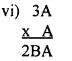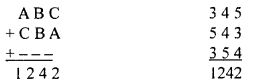# KSEEB Solutions for Class 8 Maths Chapter 1 Playing with Numbers Ex 1.2

Students can Download Maths Chapter 1 Playing with Numbers Ex 1.2 Questions and Answers, Notes Pdf, KSEEB Solutions for Class 8 Maths helps you to revise the complete Karnataka State Board Syllabus and score more marks in your examinations.

## Karnataka Board Class 8 Maths Chapter 1 Playing with Numbers Ex 1.2

Question 1.
In the following find the digits represented by the letters.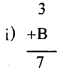Here : 3 + 4 = 7 ∴ B = 4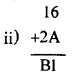Here 6 + A = 1 ∴ A = 5
6 + 5 = 11 is written in units place and 1 is carried.
∴ 1 + 1 + 2 = B
∴ B = 4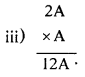Here A × A is A.
Hence A = 0, 1, 5, or 6 If A = 1 we get 21 × 1 = 21 but the product is 12A.
If A = 5 then 25 × 5 = 125.
∴ A = 5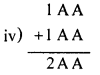Here A + A = A
Hence A = 0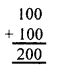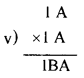Answer: Here A × A = A
Hence A = 0, 1, 5 or 6
If A = 1, then 11 × 11 = 121
∴ A = 1 and B = 2